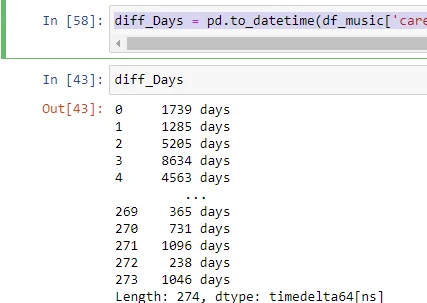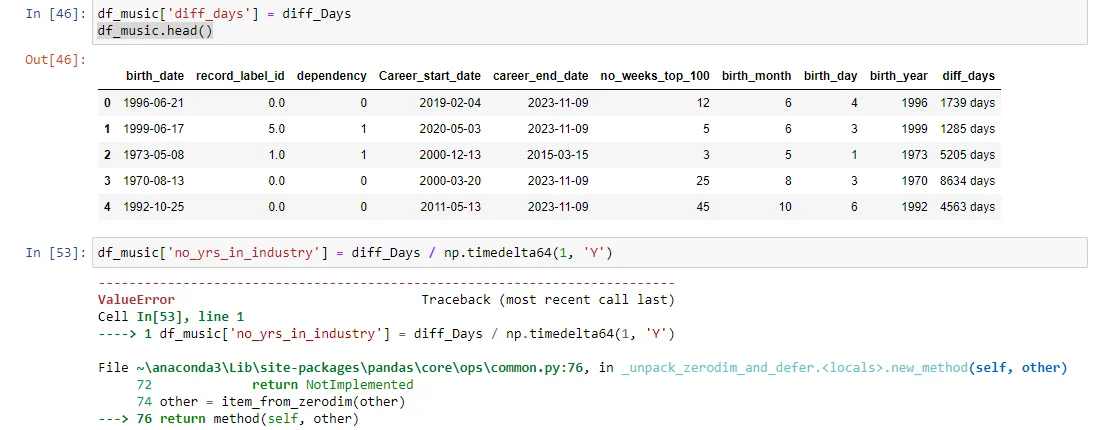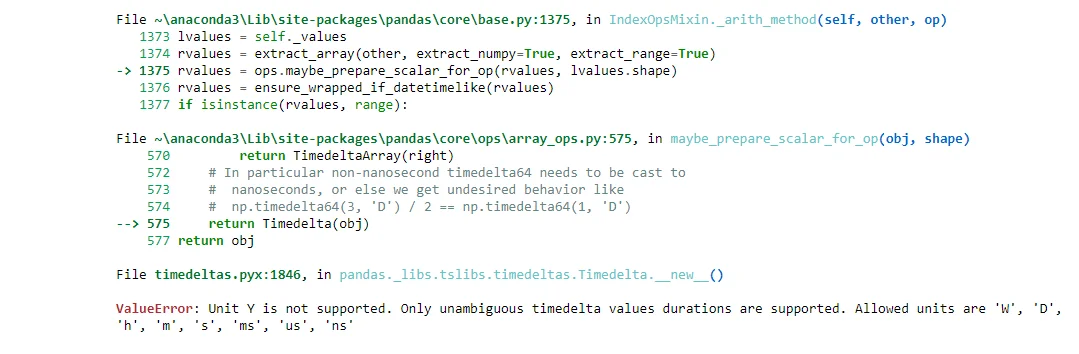10 Nov 2023

Posted on:

09 Nov 2023

0

# Clarify Practice Exam 2 - Machine Learning Section Question 7 & 8

Question 7: I executed the code below as written in the reviewed answer and i got the answer in days. Is this correct?

diff_Days = pd.to_datetime(df_music['career_end_date'], format = '%Y-%m-%d') - pd.to_datetime(df_music['Career_start_date'], format = '%Y-%m-%d')Question 8 - I proceeded to question 8 and I got this error below:  that unit 'Y' is not supported.

How do I resolve this please?Instructor
Posted on:

10 Nov 2023

0

Hi Nike!
Thanks for reaching out.

Question 7: Yes, when you subtract two `datetime` objects in Pandas, the result is a `Timedelta` object that represents the difference in days (and potentially in hours, minutes, and seconds if the time is also provided).

Question 8: The error regarding the unit 'Y' not being supported is likely because you tried to convert the `Timedelta` object into years using a method or function that doesn't recognize 'Y' as a valid unit for year. In Pandas, you can't directly convert a `Timedelta` to years because a year can vary in length (due to leap years). However, you can approximate this by dividing the number of days in the `Timedelta` by 365.25 (taking into account leap years on average):

`years = diff_Days / pd.Timedelta(days=365.25)`

This will give you the duration in years as a floating-point number. If you need to operate on a `Timedelta` object and want to extract the number of years, always ensure to handle it with a proper conversion like the above, since there's no direct 'Y' unit in Pandas time deltas.

Hope this helps.
Best,
Tsvetelin